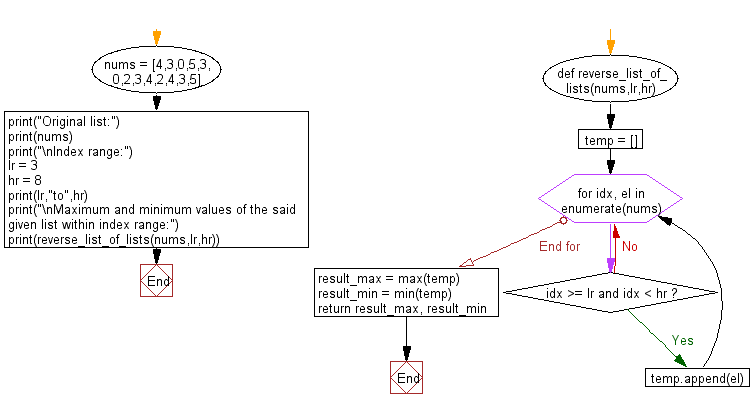﻿ Python: Find the maximum and minimum values in a given list within specified index range - w3resource# Python: Find the maximum and minimum values in a given list within specified index range

## Python List: Exercise - 151 with Solution

Write a Python program to find the maximum and minimum values in a given list within specified index range.

Sample Solution:

Python Code:

``````def reverse_list_of_lists(nums,lr,hr):
temp = []
for idx, el in enumerate(nums):
if idx >= lr and idx < hr:
temp.append(el)
result_max = max(temp)
result_min = min(temp)
return result_max, result_min
nums = [4,3,0,5,3,0,2,3,4,2,4,3,5]
print("Original list:")
print(nums)
print("\nIndex range:")
lr = 3
hr = 8
print(lr,"to",hr)
print("\nMaximum and minimum values of the said given list within index range:")
print(reverse_list_of_lists(nums,lr,hr))
```
```

Sample Output:

```Original list:
[4, 3, 0, 5, 3, 0, 2, 3, 4, 2, 4, 3, 5]

Index range:
3 to 8

Maximum and minimum values of the said given list within index range:
(5, 0)
```

Flowchart:## Visualize Python code execution:

The following tool visualize what the computer is doing step-by-step as it executes the said program:

Python Code Editor:

Have another way to solve this solution? Contribute your code (and comments) through Disqus.

What is the difficulty level of this exercise?

Test your Python skills with w3resource's quiz

﻿

## Python: Tips of the Day

Floor Division:

When we speak of division we normally mean (/) float division operator, this will give a precise result in float format with decimals.

For a rounded integer result there is (//) floor division operator in Python. Floor division will only give integer results that are round numbers.

```print(1000 // 300)
print(1000 / 300)```

Output:

```3
3.3333333333333335```